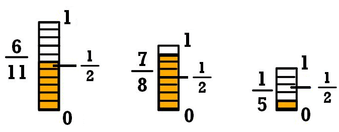# How To Round Fractions

As you probably already know, rounding numbers is a very useful operation. Even though a rounded number doesn’t have the same exact value as the real number (the one you started with), it delivers a very approximate value.

Discover the best rounding calculators.

For example, 341 rounded to the nearest hundred is 300. That is because 341 is closer in value to 300 than to 400. When rounding off to the nearest dollar, \$1.89 becomes \$2.00, because \$1.89 is closer to \$2.00 than to \$1.00.

So, today, we want to show you how you can round fractions in an easy way.

While we are well aware that many people struggle with fractions, the truth is that you just need to keep in mind that they’re no more or no less than a decimal number.

If you remember the definition of a fraction, you know that a fraction has a numerator and a denominator. Besides, it is only a different way to express a decimal number. For example, 20/5 = 4,0.

Before we show you how you can easily round fractions, we believe that it is best to review the rounding rules first.

Learn how to round numbers in C and C++.

## Rules For Rounding

While you can use different methods when you are rounding numbers, there is one general rule:

• If the number you are rounding is followed by 5, 6, 7, 8, or 9, round the number up. Example: 38 rounded to the nearest ten is 401.
• If the number you are rounding is followed by 0, 1, 2, 3, or 4, round the number down. Example: 33 rounded to the nearest ten is 30.

## How To Round Fractions

As we already said above, a fraction is simply a different way of expressing a decimal number.

So, when you are looking to round fractions, the first thing you should do is to convert the fraction into a decimal. For this article’s purposes, we will be using simple fractions so that you can easily understand. However, keep in mind that the process is exactly the same no matter which fraction you are working with.

When you are looking to round a fraction, the first question you need to ask is what are you rounding it to? After all, numbers can be rounded to the nearest ten, the nearest hundred, the nearest thousand, and so on.

Discover how to round number in javascript.

Consider the fraction 4827/1000.

As you can easily see:

4827/1000 = 4,827

So, here are some of the examples of the rounding numbers that you can get:

• 4,827 rounded to the nearest ten is 4,830
• 4,827 rounded to the nearest hundred is 4,800
• 4,827 rounded to the nearest thousand is 5,000

Looking into the banker’s rounding.

It’s important to keep in mind that all the numbers to the right of the place you are rounding to become zeros. Here are some examples with integers and decimals:

• 34 rounded to the nearest ten is 30
• 6,809 rounded to the nearest hundred is 6,800
• 1,951 rounded to the nearest thousand is 2,000

Rounding fractions works exactly the same way as rounding whole numbers. The only difference is that instead of rounding to tens, hundreds, thousands, and so on, you round to tenths, hundredths, thousandths, and so on.

• 7.8199 rounded to the nearest tenth is 7.8
• 1.0621 rounded to the nearest hundredth is 1.06
• 3.8792 rounded to the nearest thousandth is 3.879.